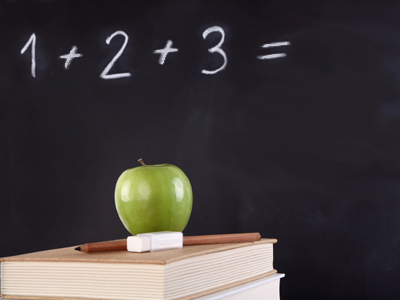1 + 2 + 3 gives the answer 6. So does 3 + 2 + 1. It doesn't matter what order you add the numbers in, you still get the same answer.

# Calculate the Total - Adding Numbers

This Math quiz is called 'Calculate the Total - Adding Numbers' and it has been written by teachers to help you if you are studying the subject at elementary school. Playing educational quizzes is a fun way to learn if you are in the 1st or 2nd grade - aged 6 to 8.

It costs only \$12.50 per month to play this quiz and over 3,500 others that help you with your school work. You can subscribe on the page at Join Us

Try this quiz to see how quickly you can find the totals.

1.
What is 3 + 9 + 4?
16
15
21
19
2.
What is the sum of 27 and 43?
70
80
60
56
Using the number bond of 7 and 3 might be useful
3.
What is the total of 2, 15 and 3?
20
19
25
15
Additions can be done in any order - putting the 15 first could be useful
4.
What is 10 + 9 + 10?
11
39
19
29
Adding the 10 and 10 up first, then adding the 9 might have helped
5.
What is 23 + 45?
75
68
65
57
Partitioning means splitting up the tens and units, making this addition easier
6.
What is 30 and 48 added together?
87
78
76
68
Adding the 30 and 40 first and then adding on the units is a good strategy here
7.
you should always put the largest number first
you should always put the smallest number first
you can add them in any order
Addition calculations can be done in any order, but subtractions cannot
8.
What is 8 + 9 + 7?
26
25
23
24
Trying to make 10 and then counting on, or using a near double of 7 + 7 could have helped
9.
What is 10 + 27 + 10?
45
37
47
54
Starting with 27 and counting up in tens is a good option to try
10.
What is the total of 7 and 26?
34
43
23
33
Starting with the larger number and counting on might be a good plan
Author:  Angela Smith